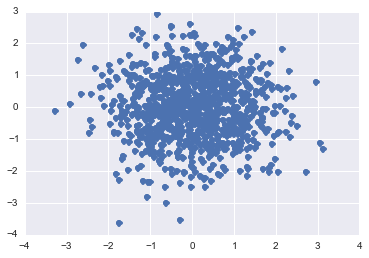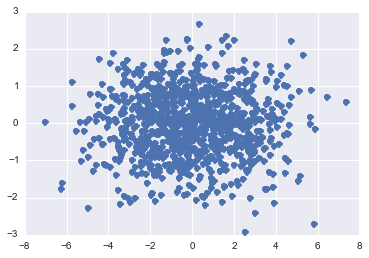# Lecture 0.5: Matrices & vectors¶

## The course¶

Now we can get to the course itself :)

## What is a matrix¶

A matrix is a two-dimensional table. Here is an example of a $3 \times 3$ matrix \begin{equation} A = \begin{pmatrix} a_{11} & a_{12} & a_{13} \\ a_{21} & a_{22} & a_{23} \\ a_{31} & a_{32} & a_{33} \end{pmatrix} \end{equation}

A vector is a $n \times 1$ vector (there are row and column vectors).

## What we can do with matrices and vectors¶

1. Add them: $a = b + c$
2. Multiply by numbers

## Matrix as a linear operator¶

Matrix is typically used to encode a linear operator: $$y = A x,$$ called matrix-by-vector product, in the index form
$$y_i = \sum_{j=1}^m A_{ij} x_j, \quad i = 1, \ldots, n.$$

## Linear dependencies¶

Many physical models are formulated as linear equations:

• Newton law $F = ma$
• Hookes law $F = kx$

which is based on the fact that if the change is small, everything can be approximated by a linear function: $$f(x + \delta x) \approx f(x) + \delta x f'(x).$$ Of course, nonlinearities may come into play, but even in this case the numerical methods linearize the problem around the current approximation. But matrices and linear dependence also play important role in data analysis as well.

## Linear dependencies in real life¶

Matrix encodes linear dependence.
Linear dependence is the simplest and often very efficient model for the data. We will give two illustrations:

• Principal component analysis
• Independent component analysis

## Demo: principal component analysis¶

One of the basic factor models, factor analysis:
$y_1, \ldots, y_P$ are vectors (data points), that are observed. We think of the as linear mixture. We generate random points on a plane and then rotate them by a certain mixture.

We generate a sequence of random points in two dimensions, and setup a rotation matrix $A$.

In :
%matplotlib inline
import matplotlib.pyplot as plt
import seaborn as sns
from sklearn.decomposition import PCA
import numpy as np

P = 1000
points = np.random.randn(P,2)
A = [[2, 1], [0, 1]]
A = np.array(A)
plt.plot(points[:, 0], points[:, 1], ls='', marker='o')

Out:
[<matplotlib.lines.Line2D at 0x1152ec810>]We can also plot the rotated points: look at how they are "skewed".

In :
R = np.dot(points, A)
#plt.plot(R[:, 0], R[:, 1], ls='', marker='o')
u, s, v  = np.linalg.svd(R, full_matrices=False)
R1 = R.dot(v)
plt.plot(R1[:, 0], R1[:, 1], ls='', marker='o')

Out:
[<matplotlib.lines.Line2D at 0x11618f210>]We can also rotate them back by finding principal components, which is equivalent to singular value decomposition (SVD). If you do not know what it is, do not worry now

In [ ]:
%matplotlib inline
u, s, v = np.linalg.svd(R, full_matrices=False)
unrotated = R.dot(v.T)
plt.plot(unrotated[:, 0], unrotated[:, 1], ls='', marker='o')
plt.plot(points[:, 0], points[:, 1], ls='', marker='x')


## Demo: Cocktail party problem¶

The linear models and the factors may have a real physical meaning. One of the most interesting illustrations is the cocktail party problem, which is defined as follows. We have a set of sources $x(t)$ (people talking) and a set of microphones.
At each microphone we record a linear mixture: $$y = A x(t) + \eta(t),$$ where $\eta(t)$ is some noise. We do not know $A$ and want to recover it.

Demo

##### Questions?¶
In :
from IPython.core.display import HTML
def css_styling():